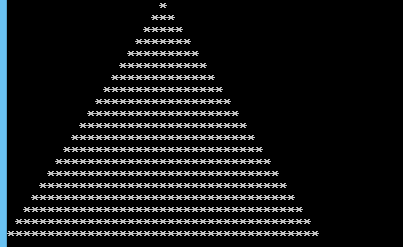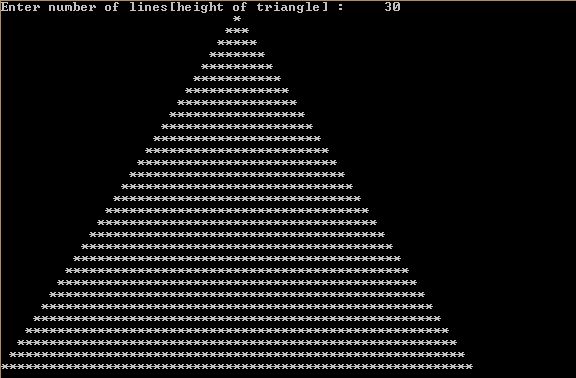## Sunday, 31 January 2016

### Cpp Program to print a triangle with stars

This is a simple Cpp Program which prints out a triangle with respect to the input given , the obtained triangle is  filled with stars.Triangle pattern with stars

Download Code

## PROGRAM :

```#include <iostream>

using namespace std;

int main()
{
int i, j, n,k;
cout << "Enter number of lines[height of triangle] : \t";
cin >> n;
for(i=1; i<=n; i++)
{
for(j=i; j<n; j++)
{
cout << " ";
}
for(k=1; k<i*2; k++)
{
cout << "*";
}
cout << "\n";
}
return 0;
}
```

## OUTPUT :Cpp Program to print a triangle with stars